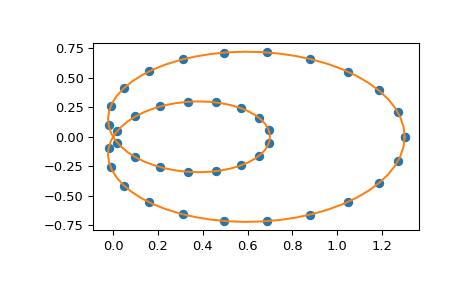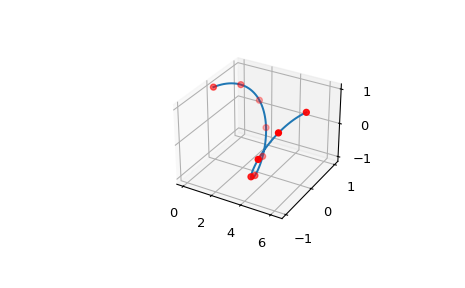scipy.interpolate.make_interp_spline¶

scipy.interpolate.make_interp_spline(x, y, k=3, t=None, bc_type=None, axis=0, check_finite=True)[source]

Compute the (coefficients of) interpolating B-spline.

Parameters
xarray_like, shape (n,)

Abscissas.

yarray_like, shape (n, …)

Ordinates.

kint, optional

B-spline degree. Default is cubic, k=3.

tarray_like, shape (nt + k + 1,), optional.

Knots. The number of knots needs to agree with the number of datapoints and the number of derivatives at the edges. Specifically, nt - n must equal len(deriv_l) + len(deriv_r).

bc_type2-tuple or None

Boundary conditions. Default is None, which means choosing the boundary conditions automatically. Otherwise, it must be a length-two tuple where the first element sets the boundary conditions at x and the second element sets the boundary conditions at x[-1]. Each of these must be an iterable of pairs (order, value) which gives the values of derivatives of specified orders at the given edge of the interpolation interval. Alternatively, the following string aliases are recognized:

• "clamped": The first derivatives at the ends are zero. This is

equivalent to bc_type=([(1, 0.0)], [(1, 0.0)]).

• "natural": The second derivatives at ends are zero. This is equivalent to bc_type=([(2, 0.0)], [(2, 0.0)]).

• "not-a-knot" (default): The first and second segments are the same polynomial. This is equivalent to having bc_type=None.

• "periodic": The values and the first k-1 derivatives at the ends are equivalent.

axisint, optional

Interpolation axis. Default is 0.

check_finitebool, optional

Whether to check that the input arrays contain only finite numbers. Disabling may give a performance gain, but may result in problems (crashes, non-termination) if the inputs do contain infinities or NaNs. Default is True.

Returns
ba BSpline object of the degree k and with knots t.

BSpline

base class representing the B-spline objects

CubicSpline

a cubic spline in the polynomial basis

make_lsq_spline

a similar factory function for spline fitting

UnivariateSpline

a wrapper over FITPACK spline fitting routines

splrep

a wrapper over FITPACK spline fitting routines

Examples

Use cubic interpolation on Chebyshev nodes:

>>> def cheb_nodes(N):
...     jj = 2.*np.arange(N) + 1
...     x = np.cos(np.pi * jj / 2 / N)[::-1]
...     return x
>>> x = cheb_nodes(20)
>>> y = np.sqrt(1 - x**2)
>>> from scipy.interpolate import BSpline, make_interp_spline
>>> b = make_interp_spline(x, y)
>>> np.allclose(b(x), y)
True

Note that the default is a cubic spline with a not-a-knot boundary condition

>>> b.k
3

Here we use a ‘natural’ spline, with zero 2nd derivatives at edges:

>>> l, r = [(2, 0.0)], [(2, 0.0)]
>>> b_n = make_interp_spline(x, y, bc_type=(l, r))  # or, bc_type="natural"
>>> np.allclose(b_n(x), y)
True
>>> x0, x1 = x, x[-1]
>>> np.allclose([b_n(x0, 2), b_n(x1, 2)], [0, 0])
True

Interpolation of parametric curves is also supported. As an example, we compute a discretization of a snail curve in polar coordinates

>>> phi = np.linspace(0, 2.*np.pi, 40)
>>> r = 0.3 + np.cos(phi)
>>> x, y = r*np.cos(phi), r*np.sin(phi)  # convert to Cartesian coordinates

Build an interpolating curve, parameterizing it by the angle

>>> from scipy.interpolate import make_interp_spline
>>> spl = make_interp_spline(phi, np.c_[x, y])

Evaluate the interpolant on a finer grid (note that we transpose the result to unpack it into a pair of x- and y-arrays)

>>> phi_new = np.linspace(0, 2.*np.pi, 100)
>>> x_new, y_new = spl(phi_new).T

Plot the result

>>> import matplotlib.pyplot as plt
>>> plt.plot(x, y, 'o')
>>> plt.plot(x_new, y_new, '-')
>>> plt.show()Build a B-spline curve with 2 dimensional y

>>> x = np.linspace(0, 2*np.pi, 10)
>>> y = np.array([np.sin(x), np.cos(x)])

Periodic condition is satisfied because y coordinates of points on the ends are equivalent

>>> ax = plt.axes(projection='3d')
>>> xx = np.linspace(0, 2*np.pi, 100)
>>> bspl = make_interp_spline(x, y, k=5, bc_type='periodic', axis=1)
>>> ax.plot3D(xx, *bspl(xx))
>>> ax.scatter3D(x, *y, color='red')
>>> plt.show()